Worksheets and No Prep Teaching Resources
Math Worksheets
Place Value Worksheets

# Ones, Tens, Hundreds, and ThousandsWorksheets, Lessons, and Printables

Place Value No Prep Book #1
Place Value No Prep Book #1 - Not Boring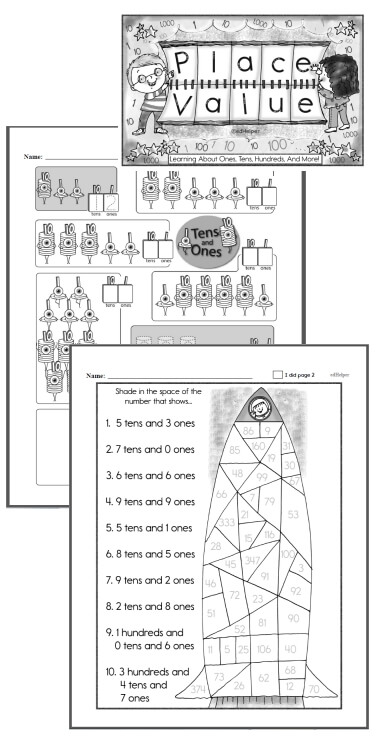Kindergarten - No Prep Place Value Book First Grade - No Prep Place Value Book Second Grade - No Prep Place Value Book

Ones, Tens, Hundreds, and Thousands
Ones, Tens, Hundreds, and Thousands - Counting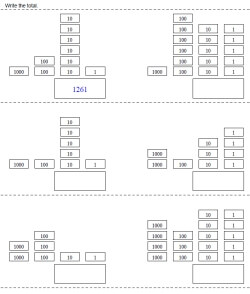Counting (first answer given) Counting

Ones, Tens, Hundreds, and Thousands - Counting Pictures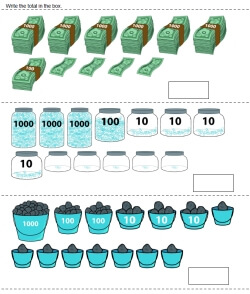Counting

Ones, Tens, Hundreds, and Thousands - Write the Place Values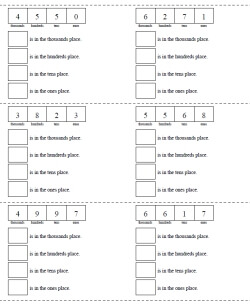Fill in all the places (text hints given for all problems) Fill in all the places (text hints given for first problem) Fill in all the places (no text hints)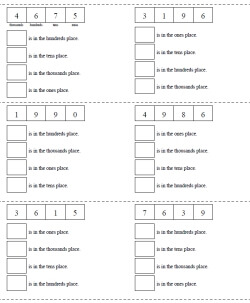Fill in all the places - random order (text hints given for first problem) Fill in all the places - random order (no text hints)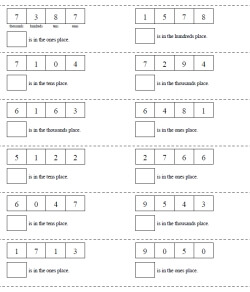Fill in one place (text hints given for first problem) Fill in one place (no text hints)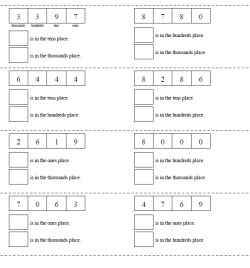Fill in two places (text hints given for first problem) Fill in two places (no text hints)

Ones, Tens, Hundreds, and Thousands - Write the Number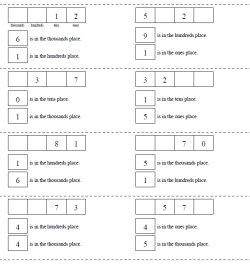Complete the Number: two places given (text hints given for first problem) Complete the Number: two places given (no text hints)

Ones, Tens, Hundreds, and Thousands - Making a Number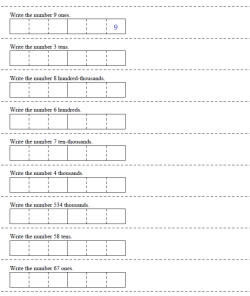Make a number from one place value (first answer given) Make a number from one place value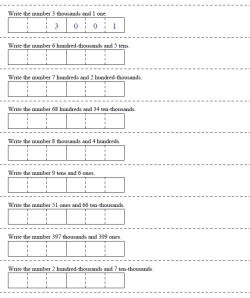Make a number by adding two place values (first answer given) Make a number by adding two place values

Place Value Number Lines

Ones, Tens, Hundreds, and Thousands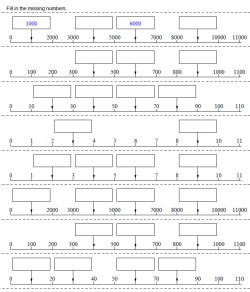Ones, tens, hundreds, and thousands number line - all start from 0 (couple answers given) Ones, tens, hundreds, and thousands number line - all start from 0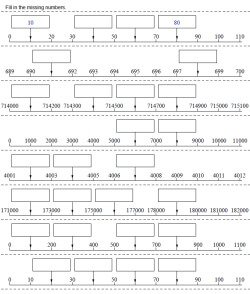Ones, tens, hundreds, and thousands number line - half of the problems start from 0 (couple answers given) Ones, tens, hundreds, and thousands number line - half of the problems start from 0 Ones, tens, hundreds, and thousands number line (couple answers given) Ones, tens, hundreds, and thousands number line

Place Value: Through Thousands
Write each number in standard form
Write each number in two other ways
Write the place and the value of the underlined digit
Fill in the missing number
Write each number in standard form (information given is mixed up)
Fill in the missing number (information given is mixed up)
Ones, Tens, and Hundreds Mixed Review
Write each number in expanded form using exponents
Write each number in standard form (includes exponents)
Review with exponents

Thousands, Hundreds, Tens, and Ones
Hundreds, Tens and Ones
Thousands, Hundreds, Tens and Ones
Thousands, Hundreds, Tens and Ones
Thousands, Hundreds, Tens, and Ones Puzzle

Rounding
Rounding Tens, Hundreds, and Thousands
Rounding Puzzle

Place Value

Have a suggestion or would like to leave feedback?